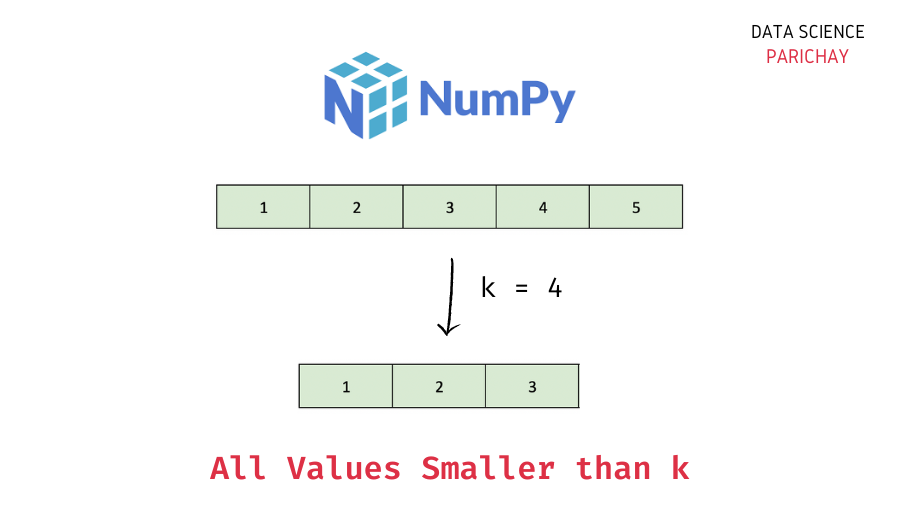# Numpy Array – Get All Values Smaller than a Given Value

The Numpy library in Python comes with a number of useful functions and methods to work with and manipulate the data in arrays. In this tutorial, we will look at how to get all the values in a Numpy array that are smaller than a given value, k with the help of some examples.

## Steps to get all the values smaller than a given value in Numpy

You can use boolean indexing to filter the Numpy array such that the resulting array contains only the elements that specify a given condition. For example, values smaller than k.

### Step 1 – Create a Numpy array

First, we will create a Numpy array that we will be using throughout this tutorial.

```import numpy as np

# create a numpy array
ar = np.array([1, 2, 3, 4, 5, 6, 7])
# display the array
print(ar)```

Output:

`[1 2 3 4 5 6 7]`

Here, we used the `numpy.array()` function to create a one-dimensional Numpy array containing some numbers.

### Step 2 – Filter the array using a boolean expression

To get all the values from a Numpy array smaller than a given value, filter the array using boolean indexing.

First, we will specify our boolean expression, `ar < k` and then use the boolean array resulting from this expression to filter our original array.

For example, let’s get all the values in the above array that are smaller than 5 (k = 5).

📚 Data Science Programs By Skill Level

Introductory

Intermediate ⭐⭐⭐

🔎 Find Data Science Programs 👨‍💻 111,889 already enrolled

Disclaimer: Data Science Parichay is reader supported. When you purchase a course through a link on this site, we may earn a small commission at no additional cost to you. Earned commissions help support this website and its team of writers.

```# values in array smaller than 5
print(ar[ar < 5])```

Output:

`[1 2 3 4]`

We get all the values in the array `ar` that are smaller than 5.

To understand what’s happening here, let’s look under the hood. Let’s see what we get from the expression `ar < 5`.

`ar < 5`

Output:

`array([ True,  True,  True,  True, False, False, False])`

We get a boolean array. The boolean values in this array represent whether a value at a particular index satisfies the given condition or not (in our case whether the element is smaller than 5 or not).

When we do `ar[ar < 5]`, we are essentially filtering the original array where the condition evaluates to `True`.

You can similarly filter a Numpy array for other conditions as well.

## Summary – Get all values smaller than a given value in a Numpy array

In this tutorial, we looked at how to get all the values in a Numpy array that are smaller than a given value. The following is a short summary of the steps mentioned –

1. Create a Numpy array (skip this step if you already have an array to operate on).
2. Use boolean indexing to filter the array for only the values that are smaller than the given value, `ar[ar < k]`.

You might also be interested in –

•